We use cookies and other tracking technologies to improve your browsing experience on our site, analyze site traffic, and understand where our audience is coming from. To find out more, please read our privacy policy.

By choosing 'I Accept', you consent to our use of cookies and other tracking technologies.

We use cookies and other tracking technologies to improve your browsing experience on our site, analyze site traffic, and understand where our audience is coming from. To find out more, please read our privacy policy.

By choosing 'I Accept', you consent to our use of cookies and other tracking technologies. Less

We use cookies and other tracking technologies... More

# Login or registerto boost this post!

Show some love to the author of this blog by giving their post some rocket fuel 🚀.

# Login or register to start working on this issue!

Engineers who find a new job through Blockchain Works average a 15% increase in salary 🚀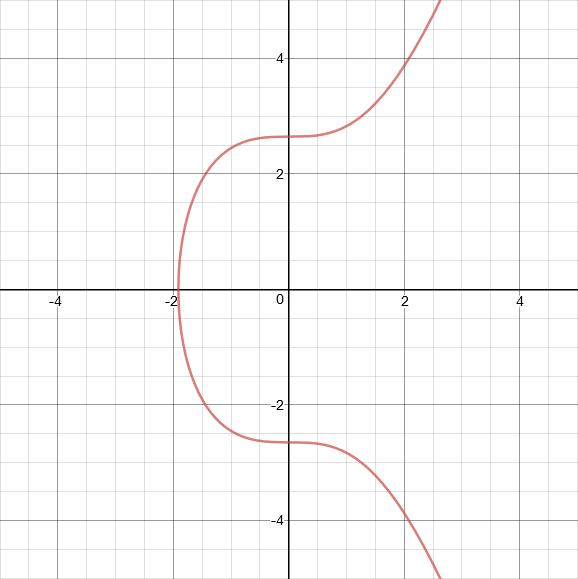# What is the math behind elliptic curve cryptography?

Hans Knutson 3 August, 2018 | 12 min read### Introduction

When someone sends bitcoin to you, they send the bitcoin to your address. If you want to spend any of the bitcoin that is sent to your address, you create a transaction and specify where your bitcoin ought to go. Such a transaction may look like:

`Transfer 5 bitcoin from 1A1zP1eP5QGefi2DMPTfTL5SLmv7DivfNa (your address) to 12c6DSiU4Rq3P4ZxziKxzrL5LmMBrzjrJX (the recipient address).`

Of course, anyone can create a transaction that looks like the one above, so if it was added to the blockchain as is and without issue, then you would be out \$30,000+ whether you like it or not. Luckily, such a transaction does not belong in the blockchain, because it is missing a valid digital signature. By adding a digital signature, you can prove that you know the private key that corresponds to the address 1A1zP1eP5QGefi2DMPTfTL5SLmv7DivfN. If you don’t know the corresponding private key, then you probably shouldn’t have been telling people to send bitcoin to you via that address since you are unable to spend any of the bitcoin sent there!

When you create a bitcoin address for yourself (or an address/account for any other cryptocurrency), you generate the private key first. From the private key, you compute the corresponding public key and by hashing that public key you get your address. Hopefully you can’t choose an address first and then determine the private key from that, otherwise you could determine the private key for any address using the same method. What is Satoshi’s address again?

### Public-key cryptography

Public keys, private keys, and digital signatures form the basic components of public-key cryptography. No matter what mathematical basis is used to implement a public-key cryptographic system, it must satisfy the following, at least for our purposes:

1. It is computationally infeasible to derive the private key corresponding to a given public key.
2. It is possible to prove that one knows the private key corresponding to a public key without revealing any useful information about the private key in the process. Furthermore, such a proof can be constructed in a way that it requires a specific message to be verified. This way, the proof forms a digital signature for that message.

One way to do public-key cryptography is with elliptic curves. Another way is with RSA, which revolves around prime numbers. Most cryptocurrencies — Bitcoin and Ethereum included — use elliptic curves, because a 256-bit elliptic curve private key is just as secure as a 3072-bit RSA private key. Smaller keys are easier to manage and work with.

### Elliptic curve cryptography

What is an elliptic curve? An elliptic curve consists of all the points that satisfy an equation of the following form:

`y² = x³+ax+b`

where 4a³+27b² ≠ 0 (this is required to avoid singular points).

Here are some example elliptic curves: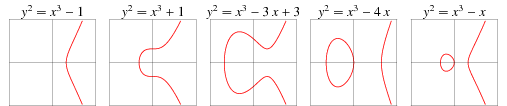Notice that all the elliptic curves above are symmetrical about the x-axis. This is true for every elliptic curve because the equation for an elliptic curve is:

y² = x³+ax+b

And if you take the square root of both sides you get:

y = ± √x³+ax+b

So if a=27 and b=2 and you plug in x=2, you’ll get y=±4, resulting in the points (2, -4) and (2, 4).

The elliptic curve used by Bitcoin, Ethereum, and many other cryptocurrencies is called secp256k1. The equation for the secp256k1 curve is y² = x³+7. This curve looks like:Satoshi chose secp256k1 for no particular reason.

You know how you can add two numbers together to get a third number? You can add two points on an elliptic curve together to get a third point on the curve.

To add two points on an elliptic curve together, you first find the line that goes through those two points. Then you determine where that line intersects the curve at a third point. Then you reflect that third point across the x-axis (i.e. multiply the y-coordinate by -1) and whatever point you get from that is the result of adding the first two points together.

Let’s take a look at an example of this. Let’s say you want to add the following two points together: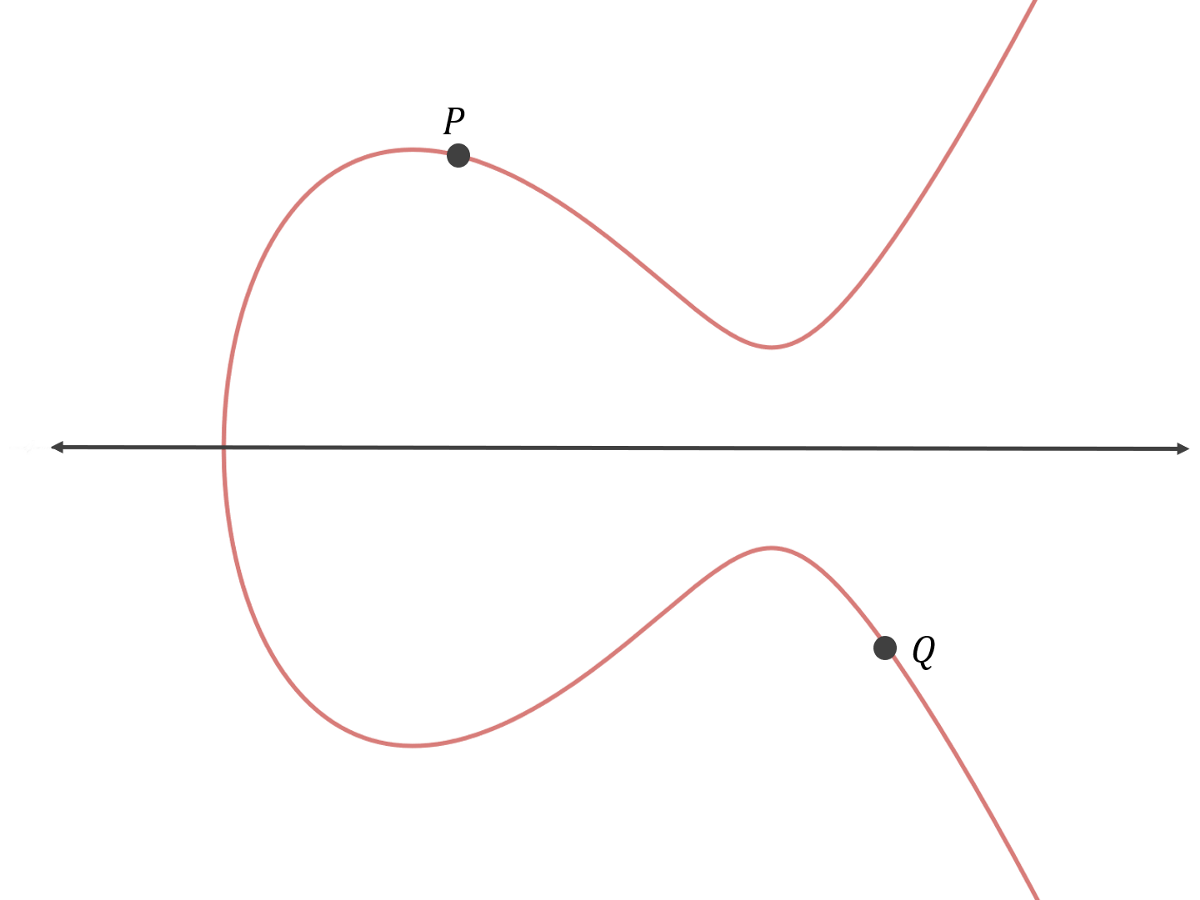First, you find the line that goes through the two points: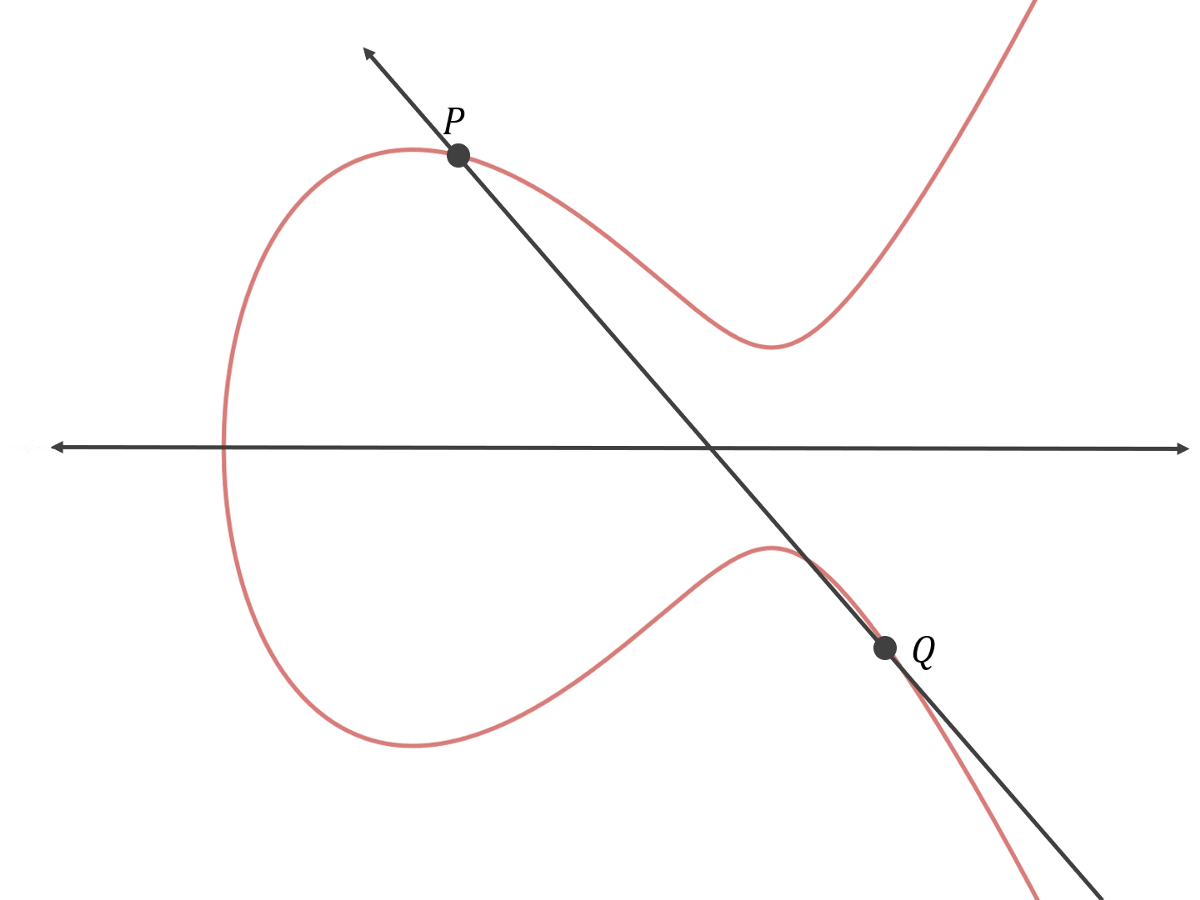Then you find the third point on the curve that the line intersects: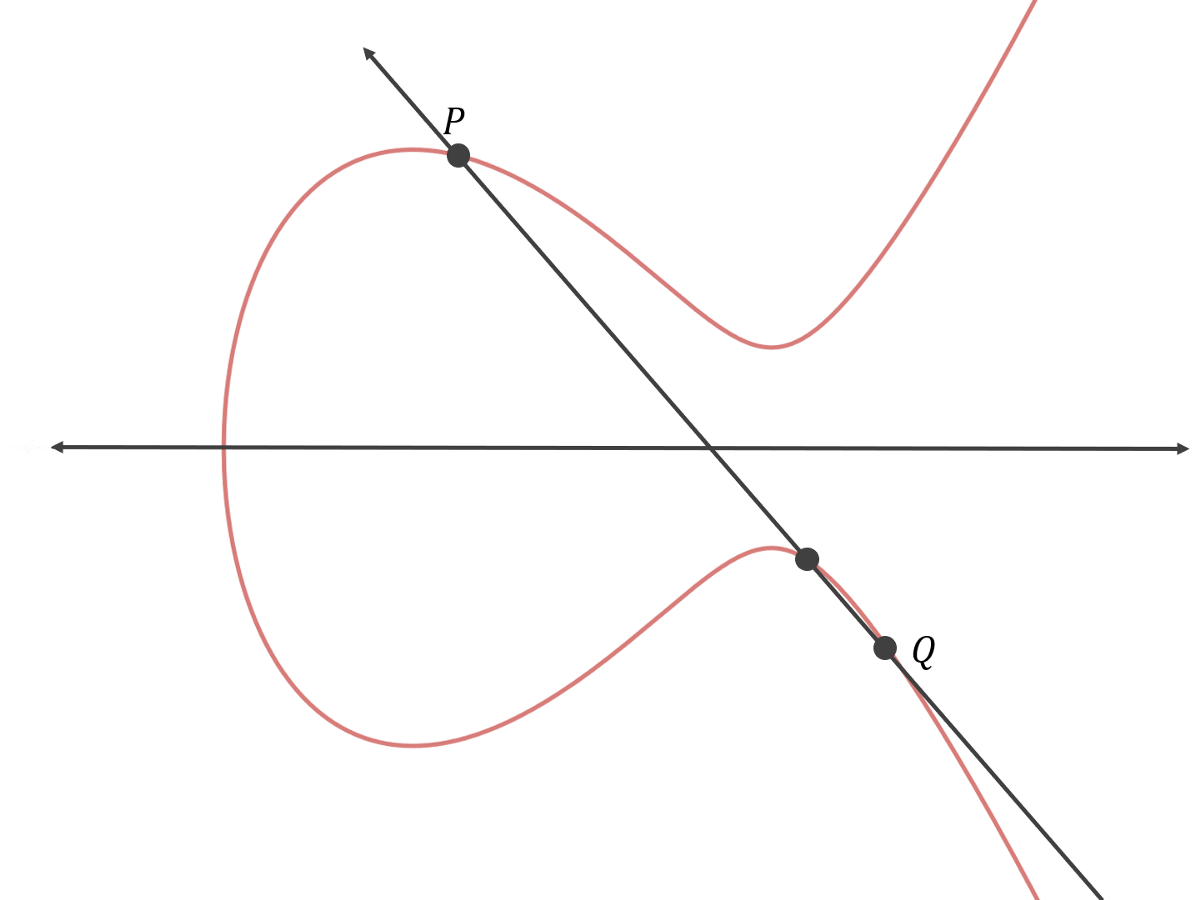Then you reflect that point across the x-axis: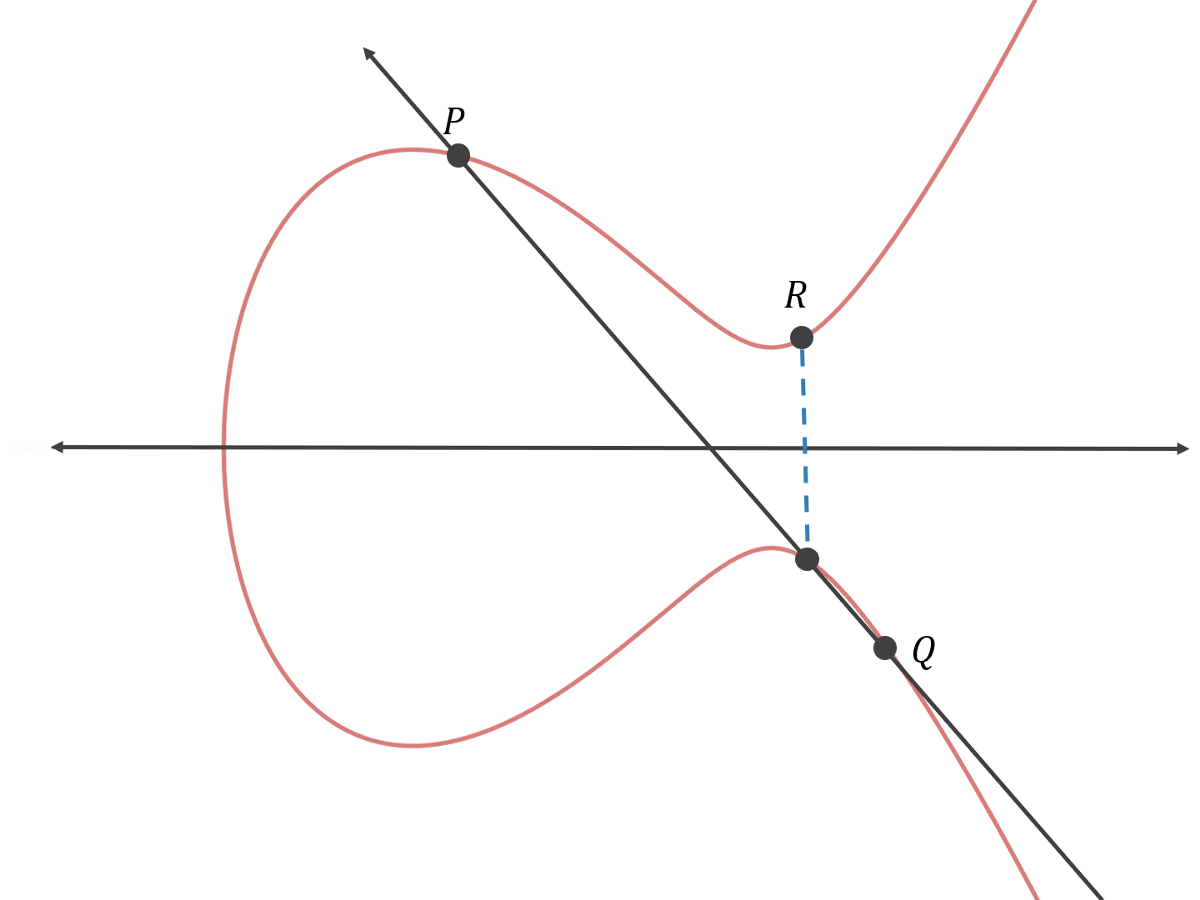Therefore, P+Q=R.

To do elliptic curve cryptography properly, rather than adding two arbitrary points together, we specify a base point on the curve and only add that point to itself.

For example, let’s say we have the following curve with base point P: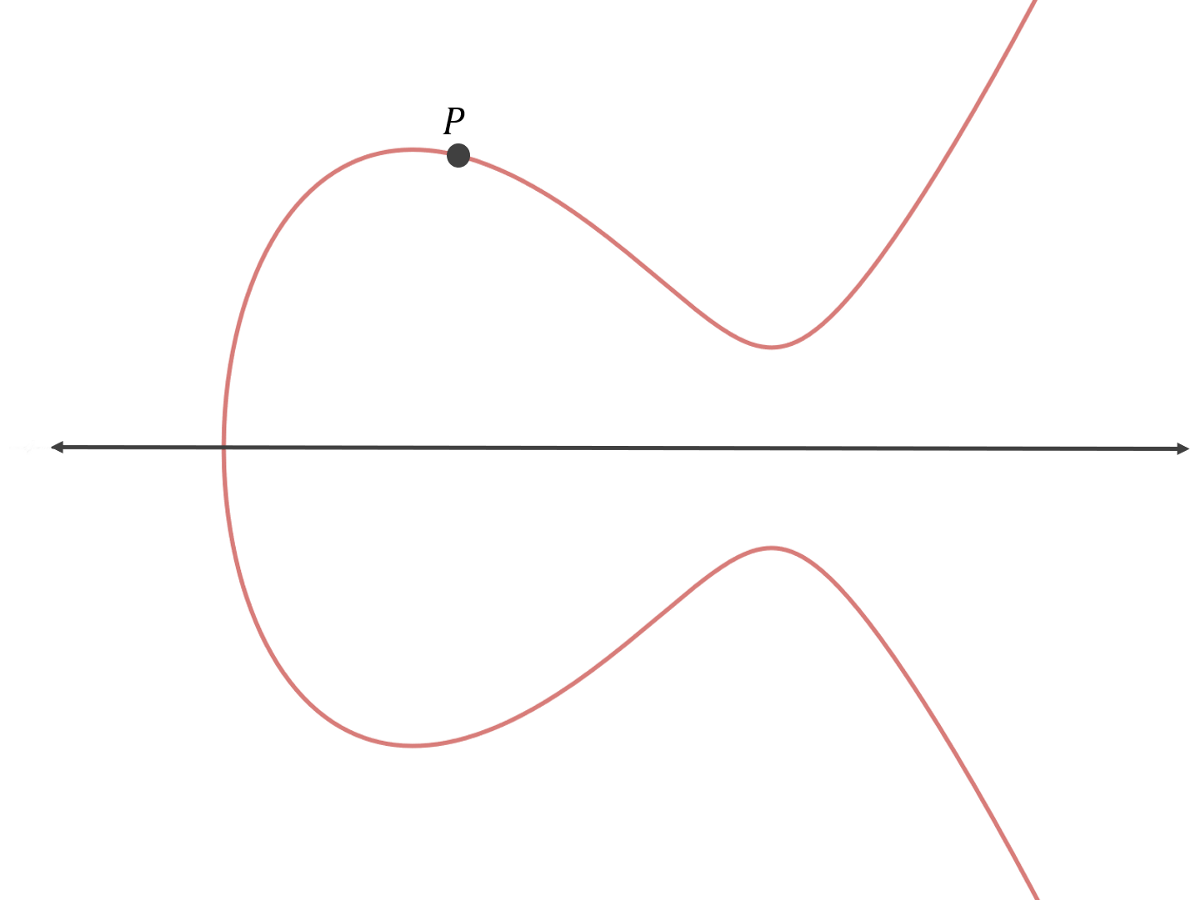Initially, we have P, or 1•P.

Now let’s add P to itself. First, we have to find the equation of the line that goes through P and P. There are infinite such lines! In this special case, we opt for the tangent line.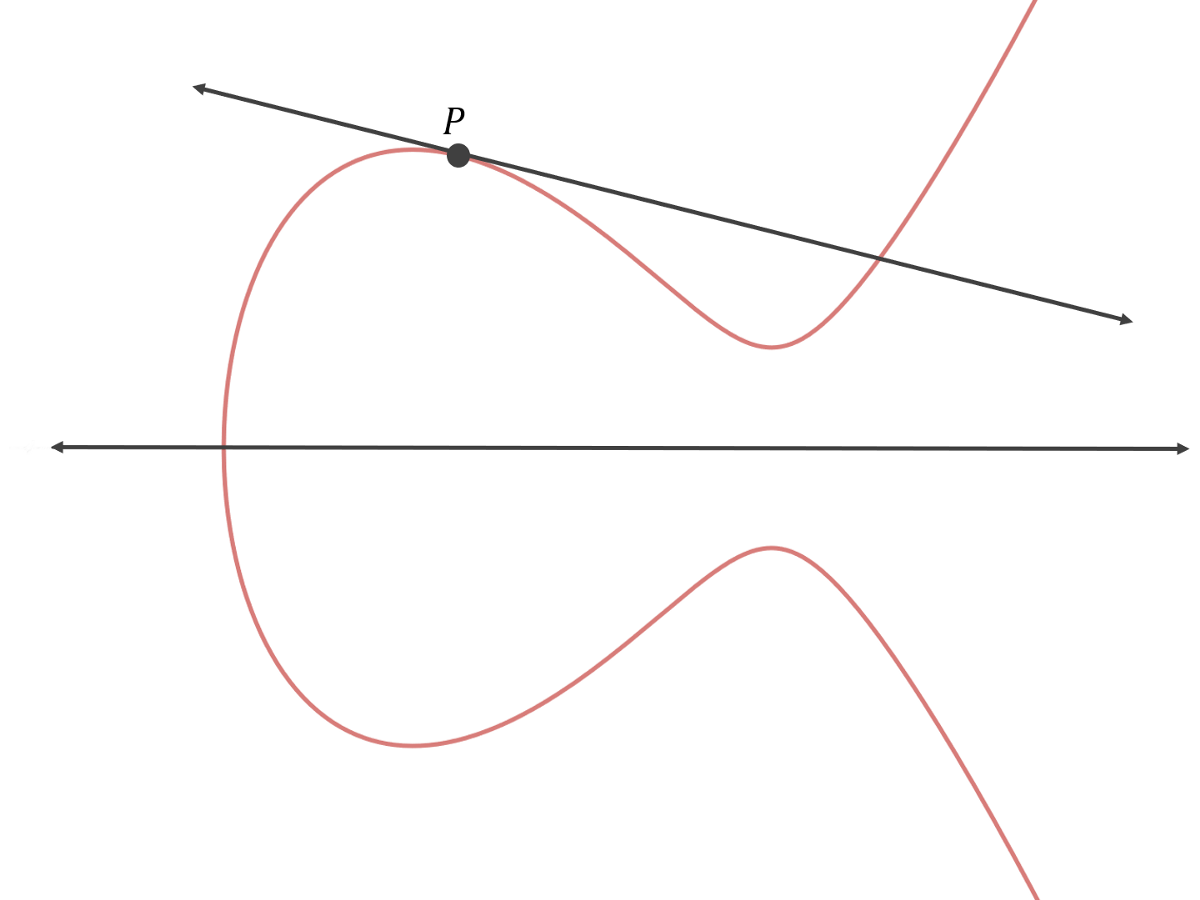Now we find the “third” point that this line intersects and reflect it across the x-axis.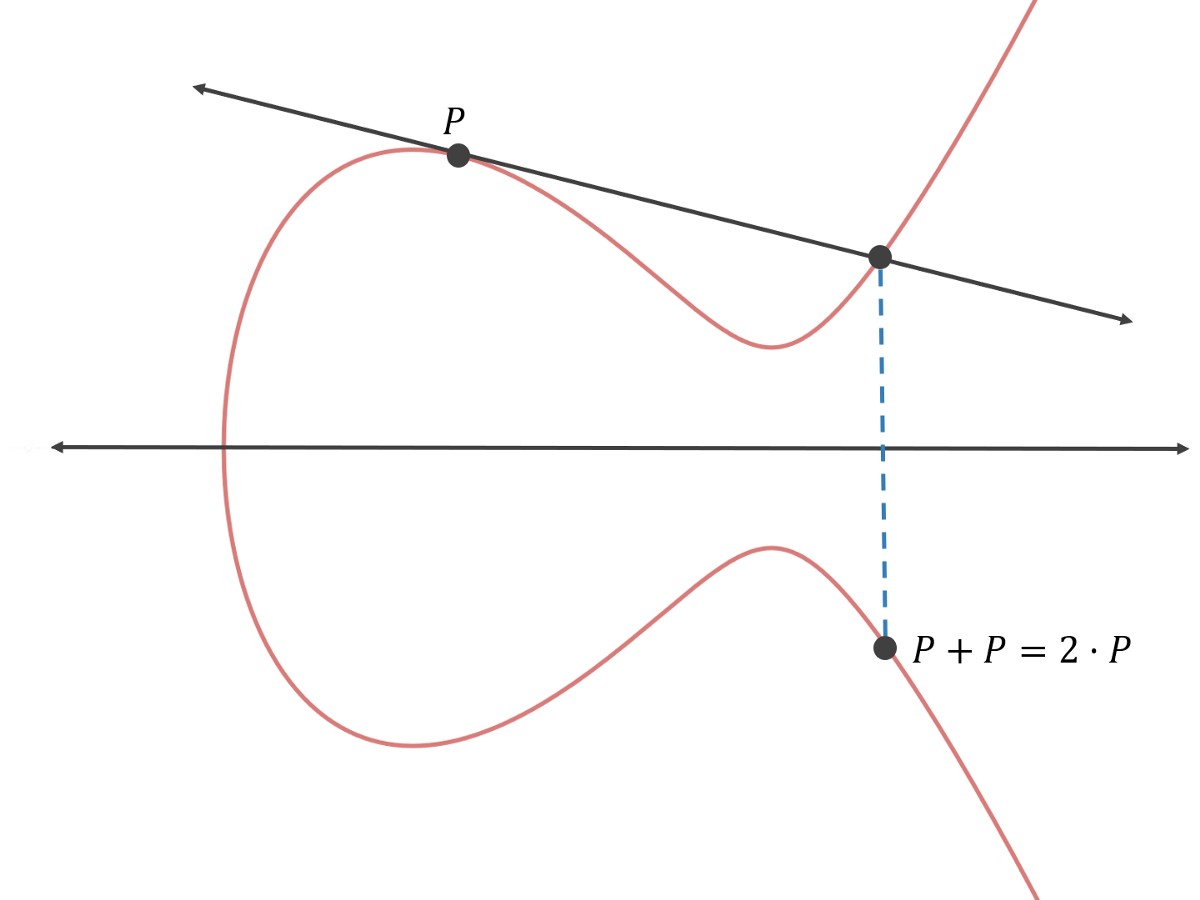Thus P added to itself, or P+P, equals 2•P.

If we add P to itself again, we’ll be computing P added to itself added to itself, or P+P+P. The result will be 3•P. To compute 3•P, we can just add P and 2•P together.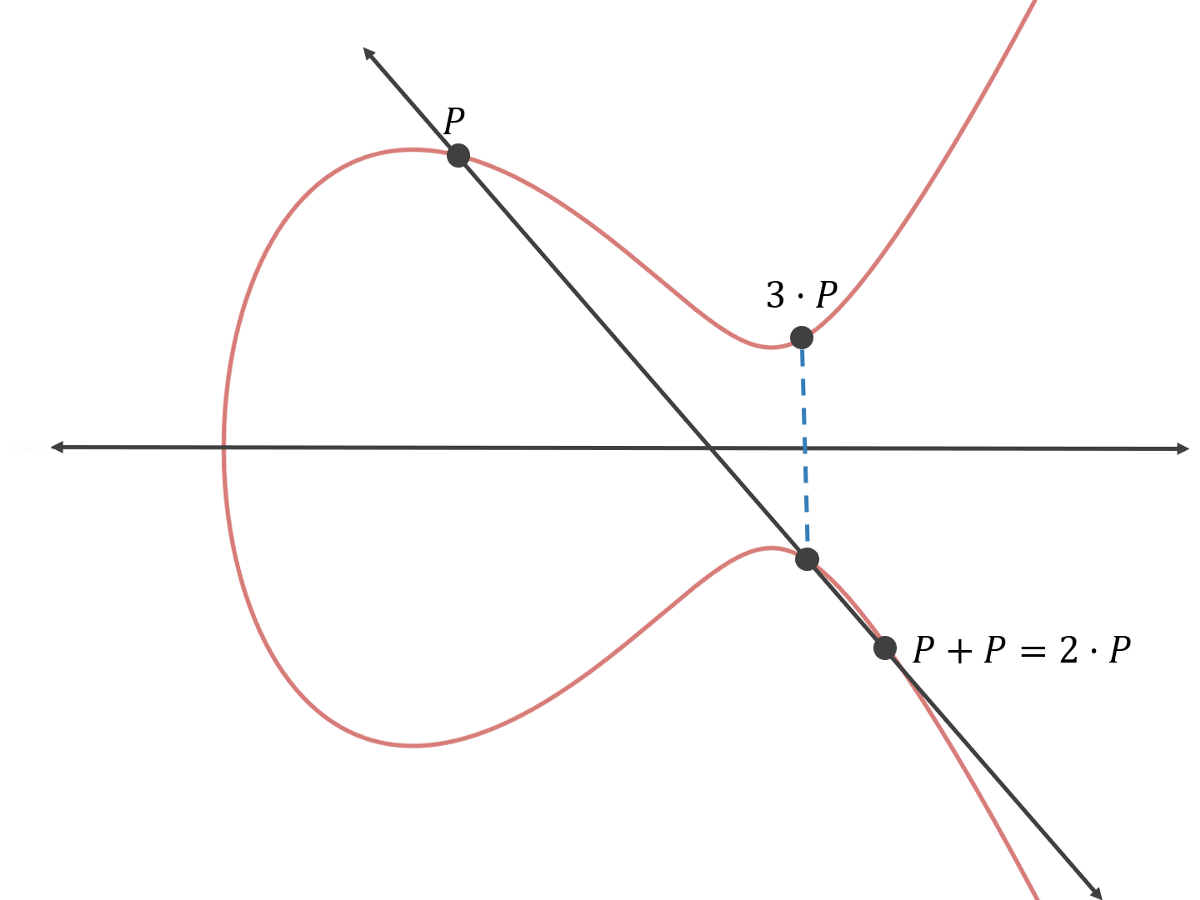We can continue to add P to itself to compute 4•P and 5•P and so on.

The base point used by secp256k1 curve has the following x- and y- coordinates:

x-coordinate: 55066263022277343669578718895168534326250603453777594175500187360389116729240

y-coordinate: 32670510020758816978083085130507043184471273380659243275938904335757337482424

In the examples above, a different base point is used so that all the point addition operations fit in a small window.

How many steps would it take to compute 10•P? It would appear to take nine steps, because 10•P is

P+P+P+P+P+P+P+P+P+P

which requires nine point addition operations.

It turns out that you can compute 10•P in just four steps. This is because the following property holds for point addition:

n•P+r•P = (n+r)•P

For example:

4•P+6•P = (4+6)•P = 10•P

Thus, the fast way to calculate 10•P is as follows:

P+P = 2•P

2•P+2•P = 4•P

4•P+4•P = 8•P

2•P+8•P=10•P

which only requires four point addition operations.

How many steps would it take to compute x•P, where x is a random 256-bit integer? In this case, x can range anywhere from 0 to 1.1579209e+77.

It turns out that computing x•P would never require more than 510 point addition operations. Here’s why. First, compute the following series:

2⁰•P, 2¹•P, 2²•P, 2³•P, 2⁴•P, 2⁵•P, 2⁶•P, … , 2²⁵⁵•P.

You can calculate the above series with 255 point addition operations, because there are 256 points, and you can get from one point to the next by adding the current point to itself. This is because 2n•P+2n•P = 2(n+1)•P. The first point, 2⁰•P, is given. 2⁰•P=1•P=P.

The next step is to find the binary expansion of x. For example, if x is 246, the binary expansion will be 2⁷ + 2⁶ + 2⁵ + 2⁴ +2² + 2¹ = 246. Then we multiply the binary expansion of x by P: 2⁷•P + 2⁶•P + 2⁵•P + 2⁴•P + 2²•P + 2¹•P. Then we simply add all these points together to get 246•P. We don’t have to compute the individual points 2¹•P, 2²•P, … , 2⁷•P since we calculated them earlier.

At most the binary expansion of x will contain 256 elements (2⁰ up to 2²⁵⁵), so we won’t ever have to add more than 256 points together, and thus, the second step will require at most 255 point addition operations. Thus, computing x•P will take at most 255+255=510 point addition operations.

### Private and public keys in elliptic curve cryptography

Let’s say I compute x•P, where x is a random 256-bit integer. The result will be some point on the curve. Let’s call that point X.

If I give you X, could you determine x? In other words, could you determine how many times I added P to itself to get the point X on the curve? Let’s assume that you know what P is and you know what curve I was using.

What if you start in the middle? You can calculate 2¹²⁸•P in 510 steps or less after all. Well, on average, x is no closer to 2¹²⁸ than it is to 0 or 2²⁵⁶-1, because x is random, so it doesn’t matter where you start — you will still have to do 2¹²⁸ point addition operations on average.

#### Private and public keys

So, because someone can’t figure out x given X, where X=x•P, it might be convenient to make x your private key and X your public key. Your private key would then be a random 256-bit integer and your public key would be the x- and y- coordinates of a point on an elliptic curve. This would satisfy the following property of private and public keys:

“It is computationally infeasible to derive the private key corresponding to a given public key.”

Furthermore, while we haven’t discussed this yet, it is possible to prove to someone that you know x, without revealing any useful information about x. That is, you can show someone that you know how many times you would have to add P to itself to get X without straight up telling them what x is.

### The update elliptic curve model

Before we get into proving to someone that you know x, we should update our elliptic curve model.

One of the problems with our current model is that x•P might result in a point whose x- and y-coordinates can’t be stored in a standard 512-bit public key. Either the x- or y-coordinate could be too long.

The solution is to define our elliptic curve over a finite field. Basically, we’ll make sure that there are only integer points and that there is a limit to how large the coordinates of a point can get.

To do this we transform

y² = x³+ax+b

to

y² mod p = (x³ + ax + b) mod p.

Where p is some prime number (p is prime to ensure that addition and multiplication operations can always be undone).

In secp256k1, p is the largest prime that is smaller than 2²⁵⁶.

Our elliptic curve now looks something like: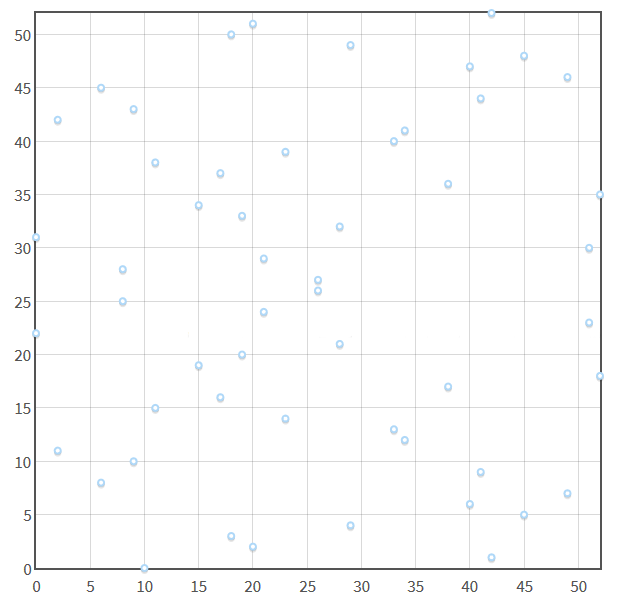Notice that there is still a horizontal line of symmetry.

So what happens when you add two points together and the line that goes between those two points goes outside of the bounds before intersecting a third point? You wrap the line around the confines!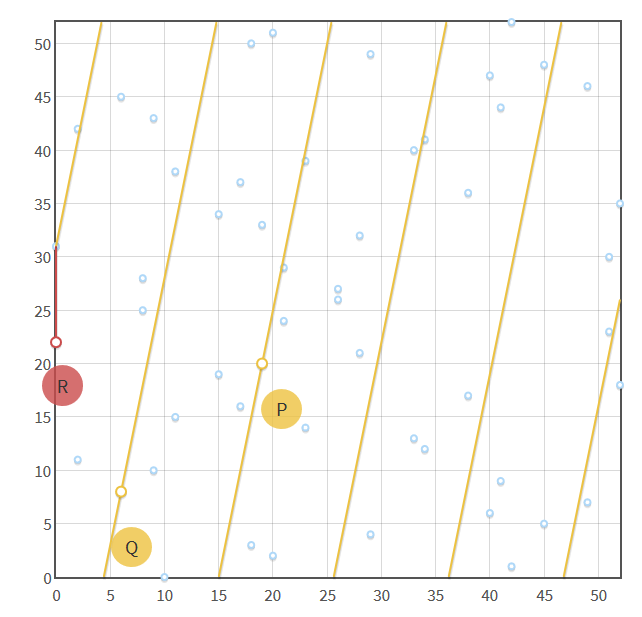In the picture above, you can see how adding P and Q together requires wrapping the line “between” P and Q around the confines several times.

Despite these changes to our model, everything we have discussed so far still applies.

### How to prove you know x

So if you compute X=x•P, where x is a random 256-bit integer, how can you prove to someone that you know the x that corresponds to X without revealing any useful information about x?

You can use the point addition property from earlier:

n•P+r•P = (n+r)•P

We will modify it slightly:

hash(m, r•P)•n•P+r•P = (hash(m, r•P)*n+r)•P

If you expand the right-hand side of the equation above, you will get the left-hand side, so the equation above holds for any m, r, and n.

So what happens if we set n•P=X? We’ll have:

hash(m, r•P)•X+r•P = (hash(m, r•P)*n+r)•P

If n•P=X, then n=x, so we have:

hash(m, r•P)•X+r•P = (hash(m, r•P)*x+r)•P

Now we’re going to make the following substitutions: R=r•P and s=hash(m,R)*x+r.

So now we have:

hash(m, R)•X+R = s•P

Okay, here’s the claim: if you can provide an m, R, and s that satisfy the above equation, then this proves that you know the x corresponding to the X in the equation, where x•P=X.

For this to be the case, the following two conditions must be met:

1. If you know x, then you should be able to provide working values for m, R, and s.
2. If you don’t know x, then you should not be able to provide working values for m, R, and s.

If you know x, you can clearly come up with working values for m, R, and s. Choose random values for m and r, then compute R=r•P and s=hash(m)*x+r. If you plug these values into hash(m,R)•X+R=s•P, then you get:

hash(m, r•P)•x•P+r•P = (hash(m, r•P)*x+r)•P

which is the equation that we said would hold earlier for any m, r, and n (x is n in this case).

What if you don’t know x? Could you come up with working values for m, R, and s? The problem is that you would have to solve hash(m,R)•X+R=s•P. Basically, you would have to find an input for a hash that has a specific hash value, which is not possible, or at least it’s not computationally feasible, because of the preimage resistance property of cryptographic hash functions.

Therefore, the only way to provide working values for m, R, and s is by computing them using x. Thus, you can prove that you know the private key, x, that goes with the public key, X, by providing values for m, R, and s that satisfy hash(m,R)•X+R=s•P.

### Do you reveal any useful information about x by proving that you know x?

If you provide working values for m, R, and s, can anything useful about x be gleaned from those values?

m and R have nothing to do with x, so those values can’t reveal any useful about x.

We know that s=hash(m,R)*x+r. Could someone compute x from s?

To do so, they would have to solve x=(s-r)/hash(m, R).

Since they don’t know r, they can’t compute x from s. They can’t obtain r from the fact that R=r•P (they are given R), because that’s the same as determining how many times P would have to be added to itself to get R, which is the same computationally infeasible problem that prevents someone from determining x from X.

s also doesn’t reveal any information about x, such as “x must be less than yadda yadda”. If r is generated randomly and we allow hash(m, R)*x+r to overflow so r can be any 256-bit integer, then the value for s is completely random, meaning s could be any 256-bit integer. A random 256-bit integer tells you just as much as about x as the GDP of New Zealand does.

### Digital signatures

m, R, and s can be used to prove that one knows the x that corresponds to X, where X=x•P. Verifying the proof requires plugging m, R, and s into hash(m,R)•X+R=s•P. Can we make it so that a specific message is required for the verification to be successful, so that the proof — m, R, and s — forms a digital signature for that message? Yes!

Let m be that specific message and R and s be the digital signature for that message. The verification process would then only be successful if the specific message, m, is plugged into the verification equation. If a different value for m is plugged in, then the left-hand side of hash(m,R)•X+R=s•P would fail to equal the right-hand side, because s was calculated using a different message.

Thus, one can prove that they know the private key, x, that corresponds to a public key, X, for a specific message, m, by providing a digital signature R and s for m.

For cryptocurrencies, the message would be the unsigned part of a transaction. If you ever look at a digital signature for a transaction, it is generally the x-coordinate of R (R is a point on the curve) concatenated with s (s is a seemingly random 256-bit integer), after it has been encoded and converted to hexadecimal.

### Conclusion

If you want to obtain a Bitcoin address or Ethereum account, you generate a random 256-bit integer x. x is then your private key. Then you compute X= x•P using the parameters for the secp256k1 curve. X will be your public key. Your public key is safe to give out and cannot be used to determine your private key. If you hash your public key, you will obtain your address.

When you want to send bitcoin or ether from your address to another address, you create a transaction. You set m to the unsigned part of that transaction, and compute R and s from that m. Then you attach R and s to the transaction. After you broadcast your transaction, any node will be able to verify that m (the unsigned part of the transaction), R, and s satisfy hash(m,R)•X+R=s•P. This of course assumes that you also include X in your transaction, since your public key cannot be determined from your address. For Ethereum, instead of providing X you provide v, which allows one to determine X from R and s.

### The end

Well, that’s the end of it! If you made it this far and understood almost everything, then congratulations! If you want a higher level explanation of elliptic curve cryptography (more mathematical), then check out this link. This is my first article, so let me know if there are any mistakes and please leave feedback.

Originally published on hackernoon.com

## Related Issues

viebel / klipse-clj
viebel / klipse-clj
• Open
• 0
• 0
• Intermediate
• Clojure
viebel / klipse
• Open
• 0
• 0
• Intermediate
• Clojure
viebel / klipse
• Started
• 0
• 1
• Intermediate
• Clojure
• \$100
viebel / klipse
• 1
• 0
• Intermediate
• Clojure
viebel / klipse
• Open
• 0
• 0
• Intermediate
• Clojure
• \$80
viebel / klipse
• Open
• 0
• 0
• Clojure
• \$80
viebel / klipse
• Started
• 0
• 2
• Clojure
• \$180
viebel / klipse
• Open
• 0
• 0
• Intermediate
• Clojure
viebel / klipse
• Started
• 0
• 2
• Intermediate
• Clojure
• \$80

### Get hired!

#### Sign up now and apply for roles at companies that interest you.Engineers who find a new job through Blockchain Works average a 15% increase in salary.